# Civil Engineering - UPSC Civil Service Exam Questions

26.

A locomotive has four pairs of driving wheels carrying an axle load of 24 x 104 N. The maximum load that can be pulled if the coefficient of friction is 1/6, is

 A. 32 x 104 N B. 16 x 104 N C. 8 x 104 N D. 4 x 104 N

Explanation:

No answer description available for this question. Let us discuss.

27.

A bar of circular cross-section of diameter D is subjected to a torque T at B as shown in the figure given. What is the angle of twist at A?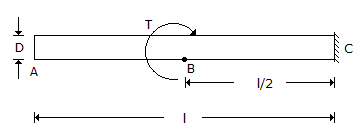A. Same as that at B B. Zero C. Twice as that at B D. Half as that at B

Explanation:

No answer description available for this question. Let us discuss.

28.

Consider the following statements regarding Plane Table surveying :
1. It is less accurate than chain surveying.
2. It is not necessary to do accurate centering of plane table for small scale survey.
3. Compass rule may be made use for adjusting the plane table transverse.
4. From the instrument station, resectors are drawn to plot the position of object in the field.
Of these statements :

 A. 1, 2 and 4 are correct B. 2, 3 and 4 are correct C. 1, 2 and 3 are correct D. 1, 3 and 4 are correct

Explanation:

No answer description available for this question. Let us discuss.

29.

A pin-jointed tower truss is loaded as shown in the given figure. The force induced in the member DF is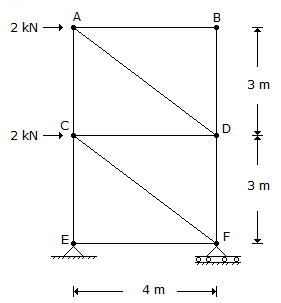A. 1.5 kN (tension) B. 4.5 kN (tension) C. 1.5 kN (compression) D. 4.5 kN (compression)

Explanation:

No answer description available for this question. Let us discuss.

30.

In a PERT network, the activity durations are given as to (optimistic), tp (pessimistic) and tm (most likely time). What is the variance of is activity ?

 A.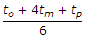B.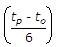C.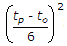D. None of the above